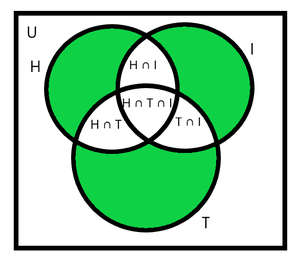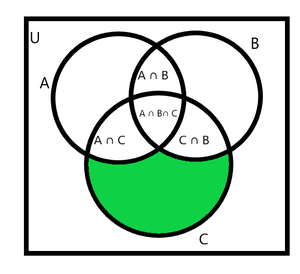Open in App
Not now

## Related Articles

• NCERT Solutions for Class 11 Maths

# Class 11 NCERT Solutions- Chapter 1 Sets – Miscellaneous Exercise on Chapter 1 | Set 2

• Last Updated : 05 Apr, 2021

### Question 11: Let A and B be sets. If A ∩ X = B ∩ X = φ and A ∪ X = B ∪ X for some set X, show that A = B

Solution:

A = A ∩ (A ∪ X) //absorption law
A = A ∩ (B ∪ X) //given that A ∩ X = B ∩ X
= (A ∩ B) ∪ (A ∩ X) //distributive law
= (A ∩ B) ∪ φ //given that A ∩ X = φ
A = (A ∩ B) …(1)
Repeating above process by taking B = B ∩ (B ∪ X)
We get B = (A ∩ B) …(2)
from (1) and (2)
A = B

### Question 12: Find sets A, B and C such that A ∩ B, B ∩ C and A ∩ C are non-empty sets and A ∩ B ∩ C = φ

Solution:

(A ∩ B) should be non-empty means atleast one element is common in between them
… same for (B ∩ C) & (A ∩ C)
A ∩ B ∩ C = φ means there should not be any element common in all the three sets A, B and C
Let A = {1,2} B = {2,3} and C = {1,3}
A ∩ B = {2}
B ∩ C = {3}
A ∩ C = {1}
and A ∩ B ∩ C = φ

### Question 13: In a survey of 600 students in a school, 150 students were found to be taking tea and 225 taking coffee, 100 were taking both tea and coffee. Find how many students were taking neither tea nor coffee?

Solution:

There are total 600 students
Let A and B represents sets of students taking tea and coffee respectively
n(A) = 150
n(B) = 225
Students taking both tea and coffee = n(A ∩ B) = 100
Students taking either tea or coffee = n(A ∪ B) = ?
By principle of inclusion-exclusion,
n(A ∪ B) = n(A) + n(B) – n(A ∩ B)
= 150 + 225 – 100
= 275
Now,
Number of students who neither take tea nor coffee = total students – Number of Students who either take tea or coffee
= 600 – 275
= 325
There are 325 students who neither take tea nor coffee

### Question 14: In a group of students, 100 students know Hindi, 50 know English and 25 know both. Each of the students knows either Hindi or English. How many students are there in the group?

Solution:

Let A and B represents sets of students who knows Hindi and English respectively
n(A) = 100
n(B) = 50
Number of students who know both languages = n(A ∩ B) = 25
It is given that each student knows either Hindi or English
Hence, Number of Students in a group = n(A ∪ B)
By principle of inclusion-exclusion,
n(A ∪ B) = n(A) + n(B) – n(A ∩ B)
= 100 + 50 – 25
= 125
Total students in the group are 125

### Question 15: In a survey of 60 people, it was found that 25 people read newspaper H, 26 read newspaper T, 26 read newspaper I, 9 read both H and I, 11 read both H and T, 8 read both T and I, 3 read all three newspapers. Find:

(i) the number of people who read at least one of the newspapers.

(ii) the number of people who read exactly one newspaper

Solution:

(i) Total number of people in survey = 60
Let H, I, T represents sets of people reading newspaper H, I and T respectively
n(H) = 25
n(I) = 26
n(T) = 26
People reading both H and I = n(H ∩ I) = 9
People reading both H and T = n(H ∩ T) = 11
People reading both T and I = n(T ∩ I) = 8
People reading all the three newspapers = n(H ∩ I ∩ T) = 3
(i) Number of people who read at least one of the newspapers is given by n(H ∪ I ∪ T)
By principle of inclusion-exclusion,
n(H ∪ I ∪ T) = n(H) + n(I) + n(T) – n(H ∩ I) – n(H ∩ T) – n(T ∩ I) + n(H ∩ I ∩ T)
= 25 + 26 + 26 – 9 – 11 – 8 + 3
= 52
Number of people who read at least one of the newspapers is 52

(ii) Take a look at following Venn diagramNumber of people who read exactly one newspaper are represented by green color in above diagram
= n(H ∪ I ∪ T) – n(H ∩ I) – n(H ∩ T) – n(T ∩ I) + (2 x n(H ∩ I ∩ T))
= 52 – 9 – 11 – 8 + (2 x 3)
= 24 + 6
= 30
Number of people who read exactly one newspaper are 30

### Question 16: In a survey it was found that 21 people liked product A, 26 liked product B and 29 liked product C. If 14 people liked products A and B, 12 people liked products C and A, 14 people liked products B and C and 8 liked all the three products. Find how many liked product C only.

Solution:

Let A, B, C represents sets of people who liked product A, B and C respectively
n(A) = 21
n(B) = 26
n(C) = 29
People who liked product A and B both = n(A ∩ B) = 14
People who liked product A and C both = n(A ∩ C) = 12
People who liked product B and C both = n(B ∩ C) = 14
People who liked all the three products = n(A ∩ B ∩ C) = 8
Take a look at following Venn diagramNumber of people who only like product C are represented by green colour
= n(C) – n(A ∩ C) – n(B ∩ C) + n(A ∩ B ∩ C)
= 29 – 12 – 14 + 8
= 11
Number of people who only like product C are 11

My Personal Notes arrow_drop_up